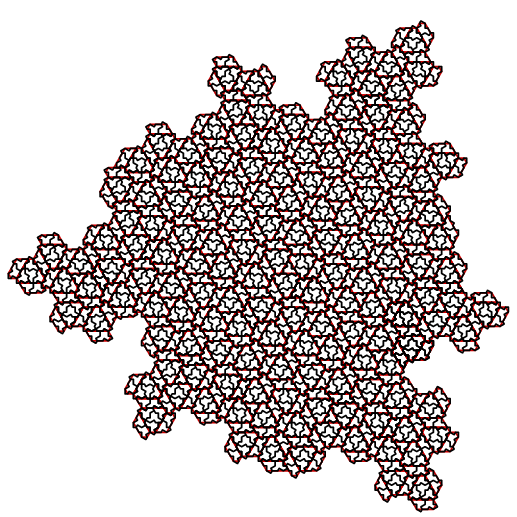# Hat Tricks

Up until recently, all known aperiodic tilings used a minimum of two shapes, and it has long been a major problem to find a tiling that uses just one. Quite recently this problem has been solved, although only with a qualification…

# Hat Tricks

Bill Casselman
University of British Columbia“But however we analyze the difference between the regular and the irregular, we must ultimately be able to account for the basic fact of aesthetic experience, the fact that delight lies somewhere between boredom and confusion.” E. H. Gombrich, in The sense of order (at the top of page 9)

Breaking News. Just as this column goes to posting, the authors of the original
construction described below have announced a new aperiodic tiling that does not use
reflected tiles.
See Kaplan’s new web page or the new arxiv submission for more information.

A tiling of the plane by a set of two-dimensional shapes is a partition of the entire plane by congruent copies of the shapes, without overlaps or gaps. One common example is the tiling by regular hexagons:Not all shapes can make a tiling. For example, the only regular polygons that tile the plane are triangles, squares, and hexagons. Regular octagons require squares to fill in, making another common pattern.These examples are periodic—they are invariant under a full discrete set of translations. More interesting from a mathematical point of view are aperiodic tilings, which are not invariant under any translations at all. Best known among these are Penrose tilings, in which pentagonal shapes play a role: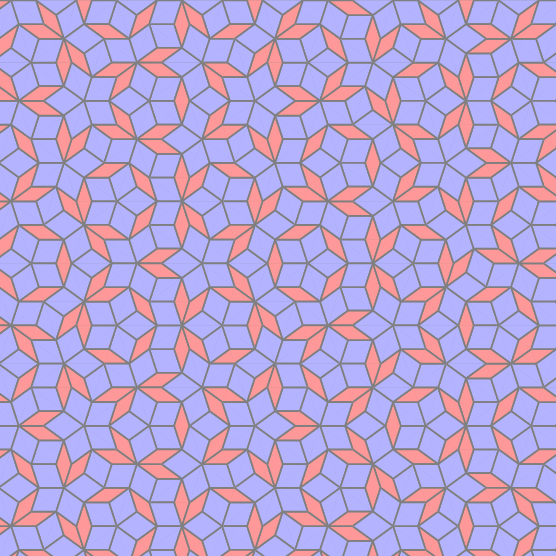Penrose tilings use two shapes, a thick and a thin rhombus.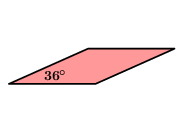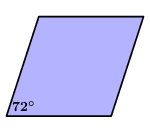Up until recently, all known aperiodic tilings used a minimum of two shapes, and it has long been a major problem to find a tiling that uses just one. Quite recently this problem has been solved, although only with a qualification: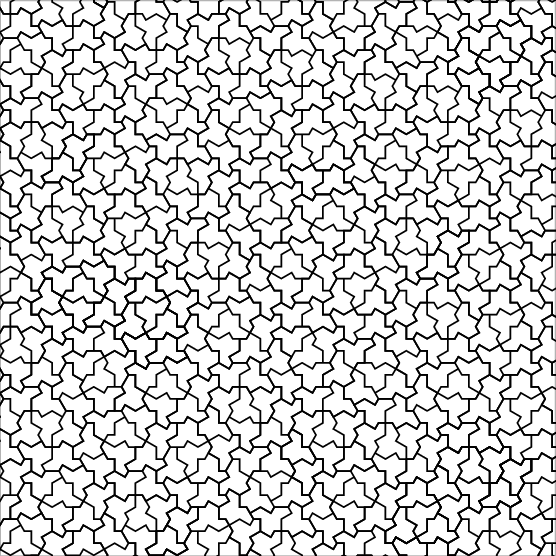The single shape that makes this tiling resembles a hat. It is in some sense a rather elementary shape, since it fits nicely into the standard hexagonal tiling of the plane: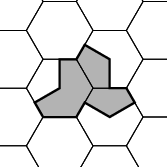This construction is due to the four authors David Smith, Joseph Samuel Myers, Craig S. Kaplan, and Chaim Goodman-Strauss. They have posted a long article about it on the arXiv, and it will presumably be eventually published in a professional journal. When it first appeared, it got a lot of media attention in places such as the Guardian and the New York Times. One thing that might have caused this attention was that the tiling was nicknamed einstein. This has nothing to do with the famous physicist. The word “ein” is German for “one”, and “Stein” is German for “stone”, incorporated for example into the word “Spielstein”, a gaming token.

However, as I have said, there is a qualification to the assertion that it uses just a single tile. That is true, but both sides of that tile are used. In the following image, the mirrored tiles are coloured: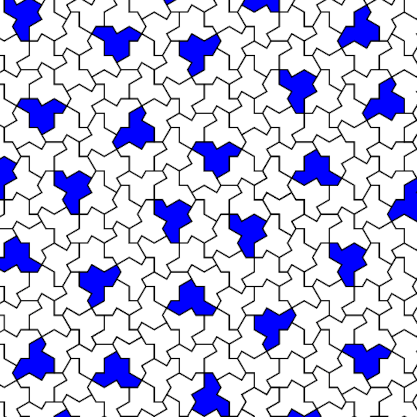In fact, the mirrored tiles play an important role in understanding the structure of this tiling. So although it is a remarkable and valuable construction, it still leaves open the problem of finding an aperiodic tiling by a single shape.

What I want to do in the rest of this essay is just to give some idea of the peculiar features of the new hat tiling. As opposed to answering questions such as: How was this construction found? Are there variants? Why, exactly, is it aperiodic? These are all quite difficult, and at least partially answered in the arxiv paper. But even carrying out my limited intention is not a simple task. In doing this, I shall be basically expanding slightly on the exposition of Smith et al.

## The structure of the tiling

Let’s look more carefully at the tiling to see if we can discern some kind of pattern. One thing that becomes quickly evident is that the neighbourhoods of the mirrored hats are all similar. The most important observation of this kind is that three unmirrored hats in this neighbourhood always form congruent triads:I’ll call these groups of four hats H-clusters.

There are now a couple of things that come to mind. One is the frequent occurrence of singleton hats inside triplets of H-clusters. Another is that near corners of the H-clusters are groups of three hats that are invariant under $120^\circ$ rotation: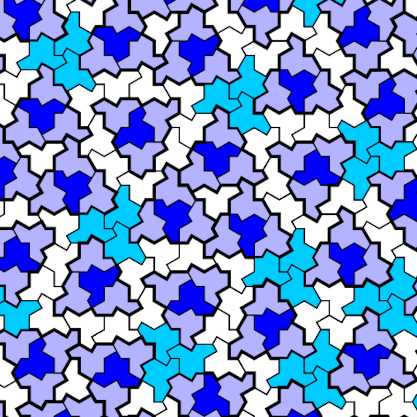These extend to larger groups of six hats, still invariant under $120^\circ$ rotation: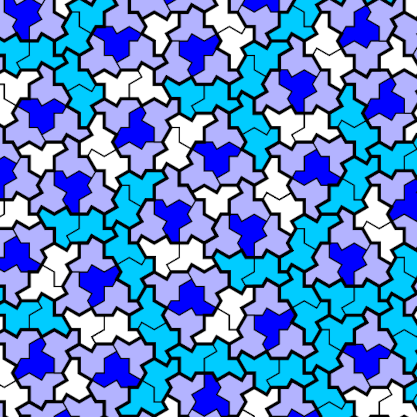What now remains unmarked is fairly simple. The unmarked tiles are either isolated hats or a couple of hats sandwiched between two H-clusters. The remarkable conclusion is that the hats in the tiling can be grouped into one of four types of clusters, and we therefore arrive at a new tiling by these four types: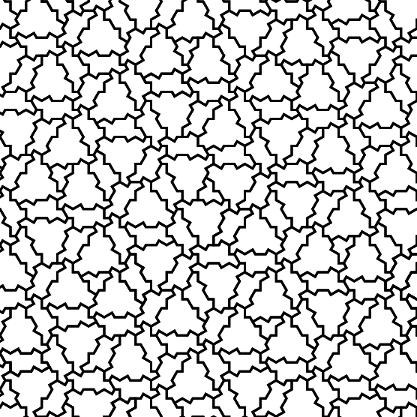Each of these clusters can be associated to a simpler figure, called a metatile. They are named, apparently arbitrarily, T, H, P, and F.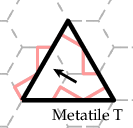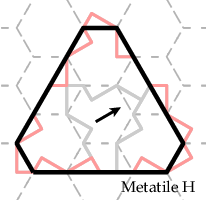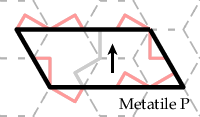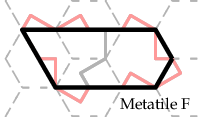Three of these shapes are invariant under some rotation, while the underlying groups of hats are not. So their orientations must be kept track of, here by arrows.

The tiling by clusters gives rise to a tiling by metatiles!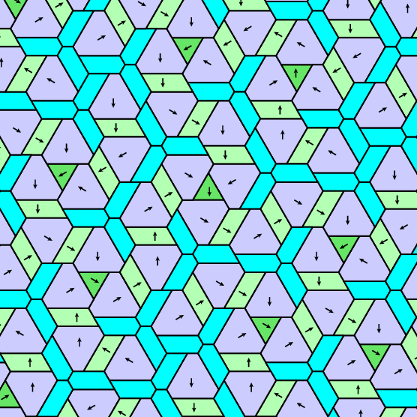This transition from hats to metatiles is the main step to the explicit construction of a tiling of the plane, although it is not at all obvious how this goes.

## Inflation and deflation

The metatiles can themselves be grouped into supertiles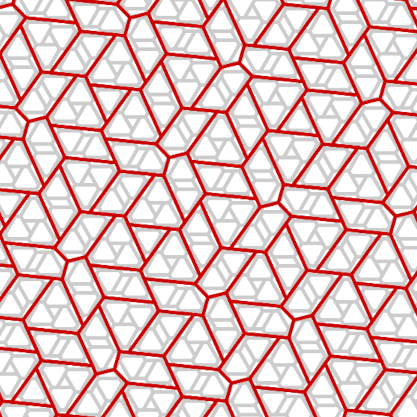… which can themselves be clustered into larger supertiles …… and so on to infinity.

The point is that if one is given just one really large supertile one can construct all of the supertiles it contains (its descendants), and then repeat this process until one reaches the bottom layer of metatiles, from which one can lay down the hats they are associated to. In this way one can construct arbitrarily large patches of hats in a consistent manner, and some very general reasoning assures you that a covering of the whole plane exists, even though of course you cannot construct it. At first it might seem extraordinary that Smith et al. came up with this scheme, but in fact it is a variation on a well known technique in the construction of aperiodic tilings—for example the Penrose tilings. Stepping up from one layer of hat/meta/super tiles to one dominating it is called inflation, and stepping down to one it dominates is called deflation. The version implemented here has one entirely new and noteworthy feature, though—the tiles in different layers are not similar shapes, but deformed a bit from descendants and parents.

## The parameters of a supertiling

So now we ask: Given a tiling by metatiles, how do you construct the tiling by supertiles that dominates it? More generally, how to step from one tiling by supertiles to the one dominating it? To the one it dominates?

All of the supertilings that occur in this business have the same general appearance—what Smith et al. call a fixed combinatorial configuration. They are characterized by a small number of parameters: lengths $a$, $x$, $y$, a vector $z_{0}$ (along with rotations), and an angle $e$.• The angle $e$ measures the tilt of the configuration.
• The $a$, $x$, $y$ are the lengths of various segments indicated in the diagram above.
• The vectors $z_{\bullet}$ indicate how the triplets of F-metatiles join together.
• Initially $a=1$, $x=1$, $y = 2$, $z_{0} = (1, 0)$, and $e=0$.
• The $g_{\bullet}$ are unit vectors in the direction of all the line segments except those parallel to the $z_{\bullet}$. Each is a rotation by $e$ of an original one. The angle $e$ is always non-positive.

All directions except the $z_{\bullet}$ are aligned with the edges of a hexagon tilted at the angle $e$. Certain nodes are vertices in both a configuration and its descendants, and the configuration is determined by these together with the vectors $z_{*}$. The supertiles of a configuration and its descendants are not similar, as the analogues for the Penrose tiling are, but Smith et al. say that asymptotically the ratio of basic lengths is $\tau = (1 + \sqrt{5})/2$. The existence of these layers of configurations, and the fact that Smith et al. discovered them, seems to me a kind of miracle.

How configurations of different levels interact, and in particular how to construct any finite sequence of configurations, ought to be roughly clear from the following diagram: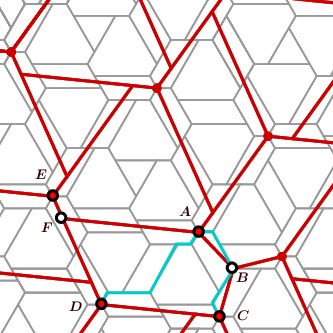With this diagram, one can use the nodes common to both layers to locate points in each, by paths in the configurations. For example, suppose we are given the configuration marked in gray, and want to find the parameters of the higher one (red). That means we are given, for example, the node $A$.

• We can then locate node $B$ by the relative translations $z_{0}$ and $-(a + 2x)\cdot g_{1}$.
• We can then go on by translations $-(a+2x)\cdot g_{2}$, $-z_{1}$ to get to $C$.
• We can similarly locate $D$.
• The segment $DE$ is then $DC$ rotated around $D$ by $120^{\circ}$, and the point $F$ is then the intersection of $DE$ with the line through $A$ parallel to $DC$.
• These points give us, with a little calculation, all the parameters $z_{\bullet}$, $a$, $x$, $y$, and $e$ for the higher layer. For example, the new $z_{1}$ is the vector $BA$, and the new $x$ is the length of the segment $EF$.

In the figure below, a few generations of F-tiles are drawn. The shapes don’t vary much and as I have said, in the limit the figure in one generation is that in the previous generation scaled by a constant related to the golden ratio. The degree of tilt starts off at $0^{\circ}$ and converges rapidly to a fixed angle, very roughly $-7^{\circ}$.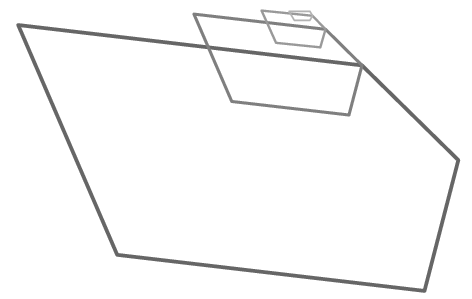Here is a different version, with the tiles rescaled so as to be comparable: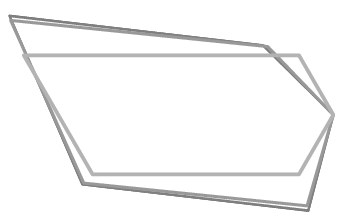Convergence is evident. It is curious, and perhaps a bit confusing, that the difference between the initial (meta)tile and the subsequent (super)tiles is so great.

## Summing up

The basic problem in drawing any aperiodic set of tiles is that construction is not local. That is to say, there is no way to start laying down tiles that does not involve some computation at a place far away. Furthermore, one can only plot tiles in a bounded region that must be fixed in advance. For hats, the process goes like this:

• Calculate the parameters of a few successive generations of supertiles by using the geometry of F-tiles to compute them.• Construct its descendants:• Then the descendants of its descendants …• Eventually, you will be looking at metatiles: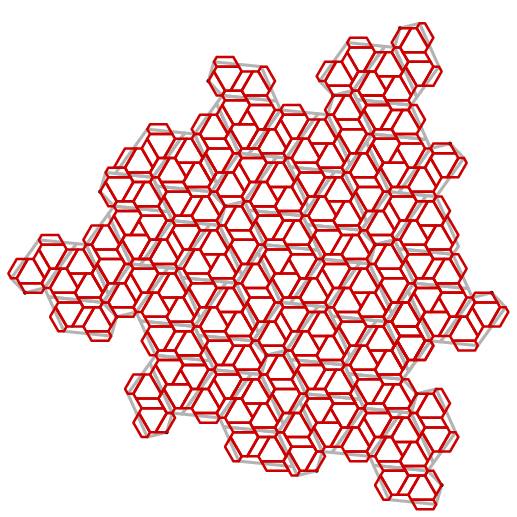• Replace them by hat clusters: